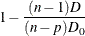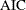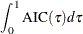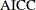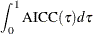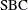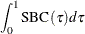# The QUANTSELECT Procedure (Experimental)

### Criteria Used in Model Selection Methods

PROC QUANTSELECT supports a variety of fit statistics that you can specify as criteria for the CHOOSE=, SELECT=, and STOP= method-options in the MODEL statement.

#### Single Quantile Effect Section

The following fit statistics are available for single quantile effect selection:

AIC

applies the Akaike’s information criterion (Akaike, 1981; Darlington, 1968; Judge et al., 1985).

AICC

applies the corrected Akaike’s information criterion (Hurvich and Tsai, 1989).

SBC

applies the Schwarz Bayesian information criterion (Schwarz, 1978; Judge et al., 1985).

SL<(LR1 | LR2)>

specifies the significance level of a statistic used to assess an effect’s contribution to the fit when it is added to or removed from a model. LR1 specifies likelihood ratio Type I, and LR2 specifies the likelihood ratio Type II. By default, the LR1 statistic is applied.

applies the adjusted quantile regression R statistic.

VALIDATE

applies the average check loss for the validation data.

Table 78.10 provides formulas and definitions for these fit statistics.

Table 78.10: Formulas and Definitions for Model Fit Summary Statistics for Single Quantile Effect Section

Statistic

Definition or Formula

n

Number of observations

p

Number of parameters including the intercept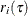Residual for the ith observation;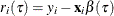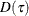Total sum of check losses;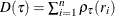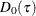Total sum of check losses for intercept-only model if intercept is a forced-in effect, otherwise for empty-model.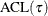Average check loss; ACL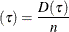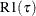Counterpart of linear regression R-square for quantile regression;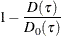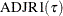Adjusted R1;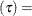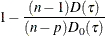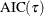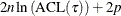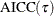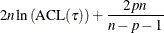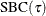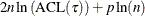#### Quantile Process Effect Section

The following statistics are available for quantile process effect selection:

AIC

specifies Akaike’s information criterion (Akaike, 1981; Darlington, 1968; Judge et al., 1985).

AICC

specifies the corrected Akaike’s information criterion (Hurvich and Tsai, 1989).

SBC

specifies Schwarz Bayesian information criterion (Schwarz, 1978; Judge et al., 1985).

specifies the adjusted quantile regression R statistic.

VALIDATE

specifies average check loss for the validation data.

Table 78.11 provides formulas and definitions for the fit statistics.

Table 78.11: Formulas and Definitions for Model Fit Summary Statistics for Quantile Process Effect Section

Statistic

Definition or Formula

D

Integral of total sum of check losses;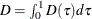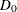Integral of total sum of check losses for intercept-only model or empty-model if the NOINT option is used;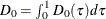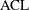Integral of average check loss; ACL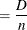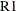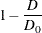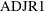Adjusted R1;